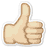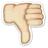Meaning Median age
What does Median age mean? Here you find 5 meanings of the word Median age. You can also add a definition of Median age yourself

# 1

10# Median age

The median age of a population is the age that divides the population into two numerically equal groups.
 Source: population-europe.eu

# 2

11# Median age

The median divides the age distribution into two equal parts: one-half of the cases falling below the median age and one-half above the median.
 Source: toxmap.nlm.nih.gov

# 3

11# Median age

Age that divides the population in two parts of equal size, that is, there are as many persons with ages above the median as there are with ages below the median.
 Source: esa.un.org

# 4

11# Median age

Median age at diagnosis is the middle value, i.e. 50% of cancer cases are diagnosed at an older age and 50% at a younger age compared to the median age.
 Source: stats.health.act.gov.au

# 5

01# median age

The median age is the age that divides the distribution of the population into two, such that half the population is below this age and half is above it.
 Source: statistics.gov.my

 Add meaning of Median age Word count:
 Name: E-mail: (* optional)

 << ICE DAM Boiling Temperature >>